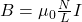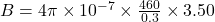## ​A 460-turn solenoid of length 30.0 cm and radius 4.00 cm carries a current of 3.50 A. What is the magnetic field strength inside the coil a

Question

​A 460-turn solenoid of length 30.0 cm and radius 4.00 cm carries a current of 3.50 A. What is the magnetic field strength inside the coil at its midpoint? What is the magnetic field strength inside the coil at its midpoint?

in progress 0
5 months 2021-08-17T07:30:18+00:00 1 Answers 4 views 0

Magnetic field at the mid point of coil is 6.74 x 10⁻³ T

Explanation:

Given :

Current flowing through the solenoid, I = 3.50 A

Length of the solenoid, L = 30 cm = 0.3 m

Number of turns in the solenoid, N = 460

Magnetic field inside the coil at its mid point is given by the relation:

B = μ₀nI    …(1)

So, equation (1) becomes:Substitute the suitable values in the above equation.B = 6.74 x 10⁻³ T